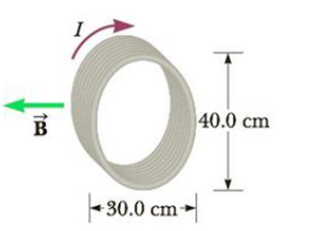Chapter 19, Problem 37P

Chapter
Section
Textbook Problem

An eight-turn coil encloses an elliptical area having a major axis of 40.0 cm and a minor axis of 30.0 cm (Fig. P19.37). The coil lies in the plane of the page and carries a clockwise current of 6.00 A. If the coil is in a uniform magnetic field of 2.00 × 10−4 T directed toward the left of the page, what is the magnitude of the torque on the coil? Hint: The area of an ellipse is A = πab, where a and b are, respectively, the semimajor and semiminor axes of the ellipse.Figure P19.37

To determine
The magnitude of the torque on the coil.

Explanation

Given info: The number of turns of the coil is 8 . The coil encloses an elliptic area. The major axis of the ellipse is 40.0cm . The minor axis of the ellipse is 30.0cm . The current flows in the plane of the page carrying clockwise current of 6.00A . The uniform magnetic field of magnitude 2.00×104T directed towards left of the page.

Explanation:

The torque applied on a circular loop is given by,

τ=nBIAsinθ

• n is the number of turns in the loop
• B is the magnetic field
• I is the current on the wire
• A is the area enclosed by the loop
• θ is the angle between the line normal to the plane of the loop and the magnetic field

Since the field is parallel to the plane the angle has to be 90° .

The area of the elliptical loop will be,

A=πab

• a is the semi major axis
• b is the semi minor axis

The major axis of the ellipse is 40.0cm . Hence,

2a=40.0cma=20.0cm

The minor axis of the ellipse is 30.0cm . Hence,

2b=30.0cma=15.0cm

Hence the torque will become,

τ=nBI(πab)sinθ

Substitute 8 for n , 20.0cm for a , 15

Still sussing out bartleby?

Check out a sample textbook solution.

See a sample solution

The Solution to Your Study Problems

Bartleby provides explanations to thousands of textbook problems written by our experts, many with advanced degrees!

Get Started

Find more solutions based on key concepts